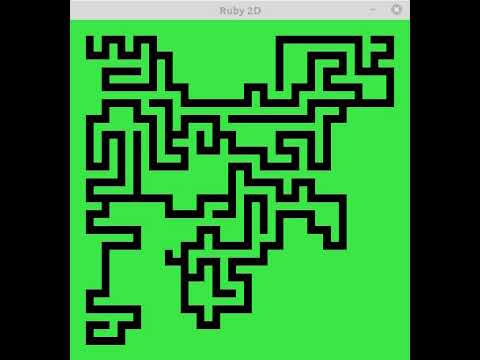# Make a digging maze with Ruby2DI tried to implement a "drilling maze" using Gem "Ruby2D". You can see the "road" gradually growing in real time. The above image is linked to a YouTube video.

## code

#### `dig_maze.rb`

``````
require "ruby2d"

L = 20    #The size of the maze
W = L * 2 + 3

Block_w = 10   #The size of one block
set width: W * Block_w, height: W * Block_w, fps_cap: 10

blocks = W.times.map {|y|
W.times.map {|x|
Square.new x: x * Block_w, y: y * Block_w,
size: Block_w, color: "green"
}
}

field = Array.new(W) {Array.new(W, 1)}
#Place a "sentinel"
field = field[-1] = Array.new(W, -1)
(1..W - 2).each {|y| field[y] = field[y][-1] = -1}

field.define_singleton_method(:show) do
each_index do |y|
self[y].each_index do |x|
end
end
end

start = [2, 2]
stack = [start]
show_stack = [start]

dig = ->(now) {
movable = []
[[1, 0], [0, -1], [-1, 0], [0, 1]].each do |dx, dy|
x = now + dx * 2
y = now + dy * 2
movable << [x, y] if field[y][x] == 1
end
if movable.empty?
return if stack.empty?
jump = stack.delete_at(rand(stack.size))
dig.(jump)
else
nxt = movable.sample
show_stack << [(now + nxt) / 2, (now + nxt) / 2]
show_stack << nxt
stack << nxt
end
}

update do
now = show_stack.shift
next unless now
field[now][now] = 0
field.show
dig.(now) if show_stack.empty?
end

show
``````

I will briefly explain the methods of Ruby2D.

--`set` Set the size of the window, etc. --`Square.new` Create an object that represents a square. Objects can be shown or hidden by adding `.add`` .remove`. --ʻUpdate` The block is updated in a certain period of time (usually 60fps). Here, it is displayed at 10 fps. --`show` Enter the main loop.

The digging itself is implemented by simple recursion. If the array variable `field` represents a maze and the value is` 0`, it means that there is a "way". There are "sentinels" around, so that there is no "road" outside.